## Scaling graphics + bounding boxes

Place to get help with not working mods / modding interface.
Pi-C
Smart Inserter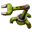Posts: 1487
Joined: Sun Oct 14, 2018 8:13 am
Contact:

### Scaling graphics + bounding boxes

I have this mod that allows to scale the size of construction and logistic bots. So far, I've only scaled the graphics:

Code: Select all

``````local function scale_position(position, scale, hr)
-- Make sure position is in correct format
position = position or { x = 0, y = 0}
if type(position) ~= "table" or table_size(position) ~= 2 then
error(serpent.line(position) .. " is not a valid position!")
end

-- Is this for the HR version?
hr = hr and 1/scale_factor or 1

local x = (position.x or position) * scale * hr
local y = (position.y or position) * scale * hr

return (position.x and position.y) and { x = x, y = y} or {x, y}
end

local function scale_image(img, scale)
if not img.scaled then
-- Set scale (defaults to 1 if not explicitly set)
img.scale = (img.scale or 1) * scale
if img.hr_version then
img.hr_version.scale = (img.hr_version.scale or .5) * scale
end

-- Set shift
img.shift = scale_position(img.shift, scale)
if img.hr_version then
img.hr_version.shift = scale_position(img.hr_version.shift, scale, "HR")
end

-- Set position
if img.position then
img.position = scale_position(img.position, scale)
if img.hr_version then
img.hr_version.position = scale_position(img.hr_version.position, scale, "HR")
end
end

-- Mark picture as scaled -- otherwise it will be scaled each time it's used!
img.scaled = true
if img.hr_version then
img.hr_version.scaled = true
end
end
end

local function recurse(tab, scale)
-- Top level may already contain an image
if tab.filename and string.match(tab.filename:lower(), "^.+%.png") then
scale_image(tab, scale)
end
for p, picture in pairs(tab) do
if type(picture) == "table" then
if picture.filename and string.match(picture.filename:lower(), "^.+%.png") then
scale_image(picture, scale)
else
recurse(picture, scale)
end
end
end
end
``````
You can see the result for the vanilla logistic bots in the left part of this picture:downscaled_bots.png (219.85 KiB) Viewed 701 times
While the graphics are smaller (50% of the original size), the bounding boxes haven't been touched and are too big. Therefore, I've added a function that scales the bounding boxes as well:

Code: Select all

``````local function scale_bounding_boxes(prototype, scale)
local bounding_box
for b, box in pairs({
"collision_box",
"drawing_box",
"hit_visualization_box",
"map_generator_bounding_box",
"selection_box",
"sticker_box",
}) do

bounding_box = prototype[box]
if bounding_box then
prototype[box] = {
scale_position(bounding_box, scale),
scale_position(bounding_box, scale),
}
end
end
end
``````
But this brings new problems:
• For a scale factor <1, the selection box has the correct dimensions, but it has an offset which didn't get scaled (see right part of the picture). Did I forget to scale some property?
• For a scale factor >1, the selection box will be too big and the game errors on loading. I've added some logging to the function scale_bounding_boxes(), here is the relevant part of the log file:

Code: Select all

``````   1.925 Script @__SmallRobots__/data-final-fixes.lua:5: Scaling bounding boxes of logistic-robot (110%)
1.925 Script @__SmallRobots__/data-final-fixes.lua:5: Looking for collision_box
1.925 Script @__SmallRobots__/data-final-fixes.lua:5: Before scaling: {{0, 0}, {0, 0}}
1.925 Script @__SmallRobots__/data-final-fixes.lua:5: After scaling: {{0, 0}, {0, 0}}
1.925 Script @__SmallRobots__/data-final-fixes.lua:5: Looking for drawing_box
1.925 Script @__SmallRobots__/data-final-fixes.lua:5: Looking for hit_visualization_box
1.925 Script @__SmallRobots__/data-final-fixes.lua:5: Before scaling: {{-0.1, -1.1000000000000001}, {0.1, -1}}
1.925 Script @__SmallRobots__/data-final-fixes.lua:5: After scaling: {{-0.11000000000000001, -1.2100000000000002}, {0.11000000000000001, -1.1000000000000001}}
1.925 Script @__SmallRobots__/data-final-fixes.lua:5: Looking for map_generator_bounding_box
1.926 Script @__SmallRobots__/data-final-fixes.lua:5: Looking for selection_box
1.926 Script @__SmallRobots__/data-final-fixes.lua:5: Before scaling: {{-0.5, -1.5}, {0.5, -0.5}}
1.926 Script @__SmallRobots__/data-final-fixes.lua:5: After scaling: {{-0.55, -1.6500000000000002}, {0.55, -0.55}}
1.926 Script @__SmallRobots__/data-final-fixes.lua:5: Looking for sticker_box!
2.028 Checksum for core: 870127790
2.028 Checksum of base: 3065294274
2.028 Checksum of SmallRobots: 1392438958
2.239 Error ModManager.cpp:1560: Error while loading entity prototype "logistic-robot" (logistic-robot): Selection box has to have distance at most 0.5 from the [0,0] point.
``````
I don't understand the error message: What exactly does "distance at most 0.5 from the [0,0] point" mean? The top left position of the unscaled selection box has y = -1.5, which is farther away from 0 than 0.5. Also, the vanilla boiler has a selection box of { {-1.5, -1}, {1.5, 1} }, where each absolute value is >0.5:prototype-browser.png (187.15 KiB) Viewed 701 times
So what the heck is wrong there?
Last edited by Pi-C on Tue Jan 24, 2023 11:10 am, edited 1 time in total.
A good mod deserves a good changelog. Here's a tutorial (WIP) about Factorio's way too strict changelog syntax!

Bilka
Factorio Staff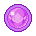Posts: 2929
Joined: Sat Aug 13, 2016 9:20 am
Contact:

### Re: Scaling graphics + bounding boxes

I like drawing, so have an image: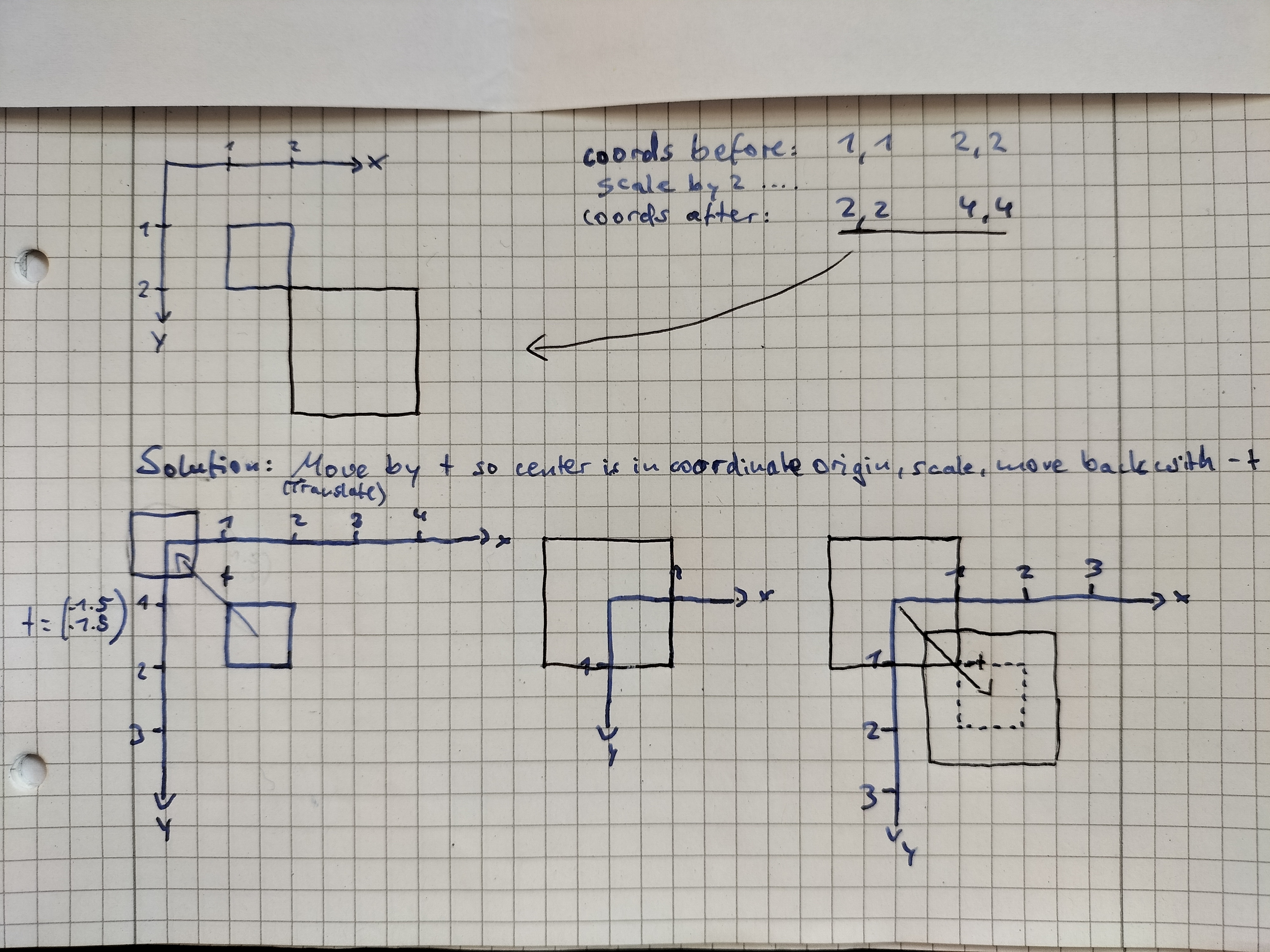In text form: Scaling also moves the box, as you noticed. The solution is to move the box (by a vector t) so the center is in the coordinate origin then scale, then move back with vector -t. You may find more info on this by searching for 2d scaling and 2d translation.

The error is related to the movement. The box needs to be close to 0,0 and due to your scale implementation moving it it's too far away.
I'm an admin over at https://wiki.factorio.com. Feel free to contact me if there's anything wrong (or right) with it.

Pi-C
Smart InserterPosts: 1487
Joined: Sun Oct 14, 2018 8:13 am
Contact:

### Re: Scaling graphics + bounding boxes

Bilka wrote:
Fri Jan 20, 2023 12:44 pm
In text form: Scaling also moves the box, as you noticed. The solution is to move the box (by a vector t) so the center is in the coordinate origin then scale, then move back with vector -t. You may find more info on this by searching for 2d scaling and 2d translation.

The error is related to the movement. The box needs to be close to 0,0 and due to your scale implementation moving it it's too far away.
Thanks for the explanation! It makes sense, and I've managed to get the selection boxes right for scale factors > 1:scale_500.png (346.69 KiB) Viewed 622 times
However, now the error occurs if the scale factor is < 1. I must somehow distinguish between scales <1 and scales >1, but everything I've tried either results in a crash or in a selection box below (instead of around) the image of the bot. For example, subtracting the vector before and adding it after scaling results in a crash, adding it first and substracting it later gives a proper box, but at the wrong position.

Here is the code I use for scaling the boxes:

Code: Select all

``````-- Get the center position of a box
local function get_center(box)
local lt = box.left_top or box
local rb = box.right_bottom or box

local x = lt.x + (rb.x - lt.x) / 2
local y = lt.y + (rb.y - lt.y) / 2

return {x = x, y = y}
end

-- Make sure all boxes are in the format {left_top, right_bottom} --> box, box
local function normalize_box(box)
if box then
local lt = box.left_top or box
local rb = box.right_bottom or box
return {
{x = lt.x or lt, y = lt.y or lt},
{x = rb.x or rb, y = rb.y or rb},
}
end
end

local function move_box(box, move_by, direction)
direction = direction or 1
if direction ~= 1 and direction ~= -1 then
error(tostring(direction).." is not a valid direction!")
end

return box and move_by and {
{ x = box.x + direction * move_by.x, y = box.y + direction * move_by.y },
{ x = box.x + direction * move_by.x, y = box.y + direction * move_by.y },
}
end

-- Apply scale_factor to bounding boxes (collision_box etc.)
local function scale_bounding_boxes(prototype, scale)
local move_box_by, must_move_box

for b, box in pairs(bounding_boxes) do
dprint("Looking for "..box.."!")
prototype[box] = normalize_box(prototype[box])
dprint("scale: "..scale)
if prototype[box] then
dprint("Original box: "..serpent.line(prototype[box]))

-- We must move the box by the vector from its center to {0, 0}, so moving the
-- box means substracting the center position from left top and right bottom.
move_box_by = get_center(prototype[box])
must_move_box = move_box_by.x ~= 0 or move_box_by.y ~= 0
dprint("Original center: "..serpent.line(move_box_by))

-- Move to {0, 0}
if must_move_box then
prototype[box] = move_box(prototype[box], move_box_by, -1)
dprint("Box after moving: "..serpent.line(prototype[box]))
dprint("Center after moving: "..serpent.line(get_center(prototype[box])))
end

-- Apply scale
prototype[box] = {
scale_position(prototype[box], scale),
scale_position(prototype[box], scale),
}
dprint("Box after scaling: "..serpent.line(prototype[box]))
dprint("Center after scaling: "..serpent.line(get_center(prototype[box])))

-- Move scaled box back
if must_move_box then
prototype[box] = move_box(prototype[box], move_box_by)
dprint("Box after moving back: "..serpent.line(prototype[box]))
dprint("Center after moving back: "..serpent.line(get_center(prototype[box])))
end
end
end
end
``````
Logging output for scales >1:

Code: Select all

``````   Looking for selection_box!
scale: 5
Original box: {{x = -0.5, y = -1.5}, {x = 0.5, y = -0.5}}
Original center: {x = 0, y = -1}
Box after moving: {{x = -0.5, y = -0.5}, {x = 0.5, y = 0.5}}
Center after moving: {x = 0, y = 0}
Box after scaling: {{x = -2.5, y = -2.5}, {x = 2.5, y = 2.5}}
Center after scaling: {x = 0, y = 0}
Box after moving back: {{x = -2.5, y = -3.5}, {x = 2.5, y = 1.5}}
Center after moving back: {x = 0, y = -1}
``````
Logging out put for scales <1:

Code: Select all

``````   Looking for selection_box!
scale: 0.5
Original box: {{x = -0.5, y = -1.5}, {x = 0.5, y = -0.5}}
Original center: {x = 0, y = -1}
Box after moving: {{x = -0.5, y = -0.5}, {x = 0.5, y = 0.5}}
Center after moving: {x = 0, y = 0}
Box after scaling: {{x = -0.25, y = -0.25}, {x = 0.25, y = 0.25}}
Center after scaling: {x = 0, y = 0}
Box after moving back: {{x = -0.25, y = -1.25}, {x = 0.25, y = -0.75}}
Center after moving back: {x = 0, y = -1}
Error while loading entity prototype "logistic-robot" (logistic-robot): Selection box has to have distance at most 0.5 from the [0,0] point.
``````
I'm obviously missing something, but having spent until late last night to figure this out, I'm afraid I don't see the forest for all the trees anymore. So if you'd have another hint, I'd really appreciate it!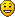A good mod deserves a good changelog. Here's a tutorial (WIP) about Factorio's way too strict changelog syntax!

Bilka
Factorio StaffPosts: 2929
Joined: Sat Aug 13, 2016 9:20 am
Contact:

### Re: Scaling graphics + bounding boxes

Pi-C wrote:
Sat Jan 21, 2023 10:06 am
For example, subtracting the vector before and adding it after scaling results in a crash,

Logging out put for scales <1:

Code: Select all

``````   Looking for selection_box!
scale: 0.5
Original box: {{x = -0.5, y = -1.5}, {x = 0.5, y = -0.5}}
Original center: {x = 0, y = -1}
Box after moving: {{x = -0.5, y = -0.5}, {x = 0.5, y = 0.5}}
Center after moving: {x = 0, y = 0}
Box after scaling: {{x = -0.25, y = -0.25}, {x = 0.25, y = 0.25}}
Center after scaling: {x = 0, y = 0}
Box after moving back: {{x = -0.25, y = -1.25}, {x = 0.25, y = -0.75}}
Center after moving back: {x = 0, y = -1}
Error while loading entity prototype "logistic-robot" (logistic-robot): Selection box has to have distance at most 0.5 from the [0,0] point.
``````
I'm obviously missing something, but having spent until late last night to figure this out, I'm afraid I don't see the forest for all the trees anymore. So if you'd have another hint, I'd really appreciate it! :-)
The box is in the correct position geometrically - same center, smaller dimensions. The error once again is the game expecting the box to be close to the entity position. The smaller box has its bottom line higher up (y = -0.75 instead of y = -0.5), so it's too far away from 0,0. I would recommend to draw the "original box" and "box after moving back" on a coordinate system if you have trouble visualizing the values.

You will have to work around the game's requirement somehow. For example you could move down the graphics (with shift) and all the boxes to be closer to the 0,0 point, however that of course means that the bot will fly lower visually.
I'm an admin over at https://wiki.factorio.com. Feel free to contact me if there's anything wrong (or right) with it.

Pi-C
Smart InserterPosts: 1487
Joined: Sun Oct 14, 2018 8:13 am
Contact:

### Re: Scaling graphics + bounding boxes

Bilka wrote:
Sat Jan 21, 2023 10:42 am
The box is in the correct position geometrically - same center, smaller dimensions. The error once again is the game expecting the box to be close to the entity position. The smaller box has its bottom line higher up (y = -0.75 instead of y = -0.5), so it's too far away from 0,0. I would recommend to draw the "original box" and "box after moving back" on a coordinate system if you have trouble visualizing the values.
Thanks, I see what you mean!
You will have to work around the game's requirement somehow. For example you could move down the graphics (with shift) and all the boxes to be closer to the 0,0 point, however that of course means that the bot will fly lower visually.
Sorry, but that's too much trouble. If I'd only wanted to scale the vanilla bots, shifting the graphics might be possible, but I want my mod to support modded bots as well, and there are too many places where things could go wrong. So I think it's best to compromise and live with a selection box below the graphics for down-scaled bots. This way, the game will at least load no matter what scale players have chosen.

I've changed the main function a bit:

Code: Select all

``````local function scale_bounding_boxes(prototype, scale)
local move_box_by, must_move_box, direction
dprint("scale: "..scale)

for b, box in pairs(bounding_boxes) do
dprint("Looking for "..box.."!")
prototype[box] = normalize_box(prototype[box])
dprint("scale: "..scale)
if prototype[box] then
dprint("Original box: "..serpent.line(prototype[box]))

-- We must move the box by the vector from its center to {0, 0}, so moving the
-- box means substracting the center position from left top and right bottom.
move_box_by = get_center(prototype[box])

-- If the center already is at position {0, 0}, we can skip moving the box!
must_move_box = move_box_by.x ~= 0 or move_box_by.y ~= 0
dprint("Original center: "..serpent.line(move_box_by))

if must_move_box then
-- For a scale > 1, we move the box towards {0, 0} (subtract), scale the
-- positions of the corners, and move the box back (add). For scales < 1, we
-- revert the process (add, scale, subtract).
direction = (scale > 1) and -1 or 1
dprint("direction: "..direction)
prototype[box] = move_box(prototype[box], move_box_by, direction)
dprint("Box after moving: "..serpent.line(prototype[box]))
dprint("Center after moving: "..serpent.line(get_center(prototype[box])))
end

-- Apply scale
prototype[box] = {
scale_position(prototype[box], scale),
scale_position(prototype[box], scale),
}
dprint("Box after scaling: "..serpent.line(prototype[box]))
dprint("Center after scaling: "..serpent.line(get_center(prototype[box])))

-- Move box back to center of scaled box
if must_move_box then
direction = direction * -1
dprint("direction: "..direction)
prototype[box] = move_box(prototype[box], move_box_by, direction)
dprint("Box after moving back: "..serpent.line(prototype[box]))
dprint("Center after moving back: "..serpent.line(get_center(prototype[box])))
end
end
end
end
``````
The log shows this for down-scaling:

Code: Select all

``````Looking for selection_box!
scale: 0.5
Original box: {{x = -0.5, y = -1.5}, {x = 0.5, y = -0.5}}
Original center: {x = 0, y = -1}
direction: 1
Box after moving: {{x = -0.5, y = -2.5}, {x = 0.5, y = -1.5}}
Center after moving: {x = 0, y = -2}
Box after scaling: {{x = -0.25, y = -1.25}, {x = 0.25, y = -0.75}}
Center after scaling: {x = 0, y = -1}
direction: -1
Box after moving back: {{x = -0.25, y = -0.25}, {x = 0.25, y = 0.25}}
Center after moving back: {x = 0, y = 0}
``````
and this for up-scaling:

Code: Select all

``````Looking for selection_box!
scale: 5
Original box: {{x = -0.5, y = -1.5}, {x = 0.5, y = -0.5}}
Original center: {x = 0, y = -1}
direction: -1
Box after moving: {{x = -0.5, y = -0.5}, {x = 0.5, y = 0.5}}
Center after moving: {x = 0, y = 0}
Box after scaling: {{x = -2.5, y = -2.5}, {x = 2.5, y = 2.5}}
Center after scaling: {x = 0, y = 0}
direction: 1
Box after moving back: {{x = -2.5, y = -3.5}, {x = 2.5, y = 1.5}}
Center after moving back: {x = 0, y = -1}
``````
The result:small+huge_bots.png (785.71 KiB) Viewed 575 times
A good mod deserves a good changelog. Here's a tutorial (WIP) about Factorio's way too strict changelog syntax!

Pi-C
Smart InserterPosts: 1487
Joined: Sun Oct 14, 2018 8:13 am
Contact:

### Re: Scaling graphics + bounding boxes

Bilka wrote:
Sat Jan 21, 2023 10:42 am
You will have to work around the game's requirement somehow. For example you could move down the graphics (with shift) and all the boxes to be closer to the 0,0 point, however that of course means that the bot will fly lower visually.
Turns out I couldn't ignore the selection boxes being too far away from the graphics after all. So I've added a check when moving the box from {0, 0} back to its original center:

Code: Select all

``````        -- Move box back to center of scaled box
if must_move_box then
direction = direction * -1
prototype[box] = move_box(prototype[box], move_box_by, direction)

-- Depending on the position of the right-bottom corner, move the box down
r_bottom = prototype[box]
move_box_by = ( (r_bottom.y < -0.5) and {x = 0, y = -0.5 - r_bottom.y}) or
( (r_bottom.y > 0.5) and {x = 0, y = 0.5 - r_bottom.y})
if move_box_by then
prototype[box] = move_box(prototype[box], move_box_by)

-- Store the final_shift! Convert it from position to vector -- if this is
-- the selection_box, we'll need this to shift the graphics!
b_data.final_shift = {move_box_by.x, move_box_by.y}
end
``````
I then pass on b_data.final_shift to the function for scaling the images and add this vector to img.shift (or {0, 0}, if img.shift doesn't exist). Finally, the selection box is around the bot, no matter what the scale factor has been set to:Scale: 50% (Atomic bots: 35%)
Bots_and_drones.png (688.66 KiB) Viewed 482 times
A good mod deserves a good changelog. Here's a tutorial (WIP) about Factorio's way too strict changelog syntax!

lyvgbfh
Fast Inserter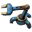Posts: 165
Joined: Fri Jul 10, 2020 6:48 pm
Contact:

### Re: Scaling graphics + bounding boxes

Pi-C wrote:
Fri Jan 27, 2023 10:05 pm
Turns out I couldn't ignore the selection boxes being too far away from the graphics after all. So I've added a check when moving the box from {0, 0} back to its original center
Something that occurred to me, and it might make your job easier here, is that if the selection box is too small the player can't reliably select the robot - especially if it's moving. Enforcing a minimum size (selection box, not graphical) may also make it easier to keep it centered and deal with the requirements around distance from [0,0]

This would also prevent occlusion of the bot graphic by the selection box like in your image above (as noted by my picky wife)

Pi-C
Smart InserterPosts: 1487
Joined: Sun Oct 14, 2018 8:13 am
Contact:

### Re: Scaling graphics + bounding boxes

lyvgbfh wrote:
Sat Jan 28, 2023 4:25 am
Pi-C wrote:
Fri Jan 27, 2023 10:05 pm
Turns out I couldn't ignore the selection boxes being too far away from the graphics after all. So I've added a check when moving the box from {0, 0} back to its original center
Something that occurred to me, and it might make your job easier here, is that if the selection box is too small the player can't reliably select the robot - especially if it's moving. Enforcing a minimum size (selection box, not graphical) may also make it easier to keep it centered and deal with the requirements around distance from [0,0]
That's what I started with, see the left part of the image posted here. I don't want the selection box to be much bigger than the image, but perhaps extending it to { {x - 0.1, y - 0.1}, {x + 0.1, y + 0.1}} for scalefactors <1 would be useful. I'll try that out.
This would also prevent occlusion of the bot graphic by the selection box like in your image above (as noted by my picky wife)
Did your picky wife note this by looking at the screenshot, or in the game? The screenshot doesn't convey that the bots are bobbing up and down, so even without my mod the bot graphics are occasionally occluded by the frame of the selection box.
A good mod deserves a good changelog. Here's a tutorial (WIP) about Factorio's way too strict changelog syntax!

lyvgbfh
Fast InserterPosts: 165
Joined: Fri Jul 10, 2020 6:48 pm
Contact:

### Re: Scaling graphics + bounding boxes

Pi-C wrote:
Sun Jan 29, 2023 11:00 am
Did your picky wife note this by looking at the screenshot, or in the game? The screenshot doesn't convey that the bots are bobbing up and down, so even without my mod the bot graphics are occasionally occluded by the frame of the selection box.
That's a good point, I hadn't considered the animations moving relative to the selection overlay.

Pi-C
Smart InserterPosts: 1487
Joined: Sun Oct 14, 2018 8:13 am
Contact:

### Re: Scaling graphics + bounding boxes

lyvgbfh wrote:
Sat Jan 28, 2023 4:25 am
[…] if the selection box is too small the player can't reliably select the robot - especially if it's moving. Enforcing a minimum size (selection box, not graphical) may also make it easier to keep it centered and deal with the requirements around distance from [0,0]
In version 1.1.5, selection_boxes with a size of x<1 and y<1 will be enlarged by 0.1 in each direction. That's still not a fixed minimum size, but I think it would work as a compromise.
A good mod deserves a good changelog. Here's a tutorial (WIP) about Factorio's way too strict changelog syntax!